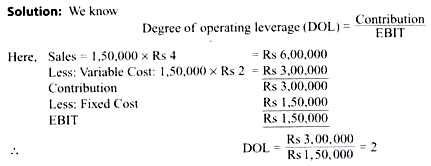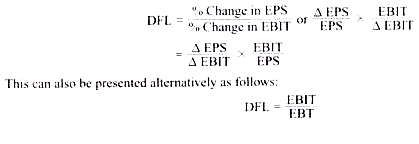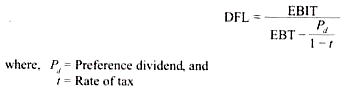# Leverage Types: Operating, Financial, Capital and Working Capital Leverage

1. Operating Leverage

Operating leverage is concerned with the investment activities of the firm. It relates to the incurrence of fixed operating costs in the firm’s income stream. The operating cost of a firm is classified into three types: Fixed cost, variable cost and semi-variable or semi-fixed cost. Fixed cost is a contractual cost and is a function of time. So it does not change with the change in sales and is paid regardless of the sales volume.

Variable costs vary directly with the sales revenue. If no sales are made variable costs will be nil. Semi-variable or semi-fixed costs vary partly with sales and remain partly fixed. These change over a range of sales and then remain fixed. In the context of operating leverage, semi-variable or semi-fixed cost is broken down into fixed and variable portions and is merged accordingly with variable or fixed cost. Investment decision goes in favor of employing assets having fixed costs because fixed operating costs can be used as a lever.

With the use of fixed costs, the firm can magnify the effect of change in sales on change in EBIT. Hence the firm’s ability to use fixed operating costs to magnify the effects of changes in sales on its earnings before interest and taxes is termed as operating leverage. This leverage relates to variation in sales and profit. Operating leverage is measured by computing the Degree of Operating Leverage (DOL). DOL expresses operating leverage in quantitative terms.

The higher the proportion of fixed operating cost in the cost structure, higher is the degree of operating leverage. The percentage change in the earnings before interest and taxes relative to a given percentage change in sales and output is defined as the DOL. Therefore,It is an interesting fact that a change in the volume of sales leads to a proportionate change in the operating profit of a firm due to the ability of the firm to use fixed operating costs. The value of degree of operating leverage should be greater than 1. If it is equal to 1, it can be said that operating leverage does not exist.

Example

Calculate the degree of operating leverage from the following data:

Sales: 1, 50,000 units at Rs 4 per unit.

Variable cost per unit Rs 2.

Fixed cost Rs 1, 50,000.

Interest charges Rs 25,000.1. Financial Leverage

Financial leverage is mainly related to the mix of debt and equity in the capital structure of a firm. It exists due to the existence of fixed financial charges that do not depend on the operating profits of the firm. Various sources from which funds are used in financing of a business can be categorized into funds having fixed financial charges and funds with no fixed financial charges. Debentures, bonds, long-term loans and preference shares are included in the first category and equity shares are included in the second category.

Financing decision goes in favour of employing funds having fixed financial charges because it can be used as a lever. Financial leverage results from the existence of fixed financial charges in the firm’s income stream. With the use of fixed financial charges, a firm can magnify the effect of change in EBIT on change in EPS. Hence financial leverage may be defined as the firm’s ability to use fixed financial charges to magnify the effects of changes in EBIT on its EPS.

The higher the proportion of fixed charge bearing fund in the capital structure of a firm, higher is the Degree of Financial Leverage (DFL) and vice-versa. Financial leverage is computed by the DFL. DEL expresses financial leverage in quantitative terms. The percentage change in the earning per share to a given percentage changes in earnings before interest and taxes is defined as Degree of Financial Leverage (DFL). ThereforeA firm is said to be highly financially leveraged if the proportion of fixed interest bearing securities, i.e. long term debt and preference share capital in the capital structure is higher in comparison to equity share capital. Like operating leverage, the value of financial leverage must be greater than 1. It is to be noted here that if the preference share capital is given in the problem the degree of financial leverage shall be computed by using the following formulaExample

Calculate the degree of financial leverage from the following information: Capital structure: 10,000, Equity Shares of Rs 10 each Rs 1, 00,000.

5,000, 11 % Preference Shares of Rs 10 each Rs 50,000.

9% Debentures of Rs 100 each Rs 50,000.

The EBIT of the company is Rs 50,000 and corporate tax rate is 45%.1. Combined Leverage

A firm incurs total fixed charges in the form of fixed operating cost and fixed financial charges. Operating leverage is concerned with operating risk and is expressed quantitatively by DOL. Financial leverage is associated with financial risk and is expressed quantitatively by DFL. Both the leverages are concerned with fixed charges. If we combine these two we will get the total risk of a firm that is associated with total leverage or combined leverage of the firm. Combined leverage is mainly related with the risk of not being able to cover total fixed charges.

The firm’s ability to cover the aggregate of fixed operating and financial charges is termed as combined leverage. The percentage change in EPS to a given percentage change in sales is defined as Degree of Combined Leverage (DCL). DCL expresses combined leverage in quantitative terms. The higher the proportion of fixed operating cost and financial charges, higher is the degree of combined leverage. Like other two leverages the value of combined leverage must be greater than 1.

DCL can be computed in the following manner:Example

X Limited has given the following information1. Working Capital Leverage

Investment in working capital has a significant impact on the profitability and risk of a business. A decrease in investment in current assets will lead to an increase in the profitability of the firm and vice versa. This is due to the fact that current assets are less profitable in comparison to fixed assets. Decrease in investment in current assets also increases the volume of risk. Risk and returns are directly related.

Therefore as risk increases, profitability of firm tends to increase. Thus Working Capital Leverage (WCL) may be defined as the ability of the firm to magnify the effects of change in current assets— assuming current liabilities remain constant—on firm’s Return on Investment (ROI).

Hence WCL may be computed as:Example

From the information given below, compute the working capital leverage.

Total assets: Rs 15, 00,000

Current assets: Rs 5, 00,000

Increase in current assets: Rs 1, 00,000.## 2 thoughts on “Leverage Types: Operating, Financial, Capital and Working Capital Leverage”

error: Content is protected !!